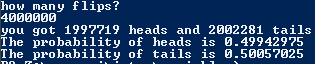# Final

## Code

```    /// Gian Adoremos
/// Period: 7
/// Program Name: Final
/// File Name: DisplayProbability.java
/// Date Finished: 11/19/2015

import java.util.Random;
import java.util.Scanner;

public class DisplayProbability
{
public static void main(String[] args)
{
Scanner key = new Scanner(System.in);
Random r = new Random();
int d1;
int numberOfHeads=0,numberOftails=0, flips;//needed for math at end

System.out.println("how many flips?");
flips = key.nextInt();
int rflips= flips;
if (flips < 1 ||flips>2100000000)//easly makes sure the number is in usable bounds
else
{
while (rflips>0)
{
d1 = r.nextInt(2);
if (d1==1)
{
}
else
{
numberOftails++;
}
rflips= rflips-1;

}